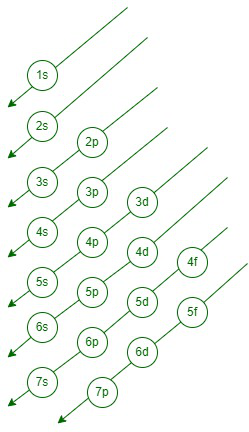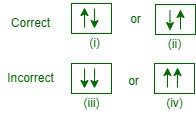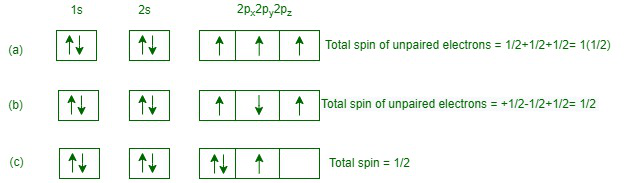GeeksforGeeks App
Open AppBrowser
Continue

## Related Articles

• CBSE Class 11 Chemistry Notes

# Filling of Orbitals in Atom

The defined structure and basic units of matter of an element are called atoms. Because the initial atom was supposed to be the tiniest item in the cosmos that could not be separated, the word “atom” derives from a Greek word that means “indivisible.” The structure of an atom consists of a nucleus, in which protons and neutrons are present. The negatively charged particles called electrons revolve around the centre of the nucleus.

### Filling of Orbitals in Atom

The filling of electrons into the orbitals of distinctive atoms takes place consequently to the succeeding three ground rules-

Aufbau Principle

The electrons in different orbitals are filled in the increasing order of their energy, i.e. the orbital with the lowest energy will be filled first and the orbital with the highest energy will be filled first.

The word ‘Aufbau’ in German means ‘Building up’. Formation of orbitals means filling orbitals with electrons. The theory states as follows-

• In the ground state of atoms, the orbitals are loaded into the arrangement of their accelerating energies. In dissimilar expressions, electrons dissociate from the lowest energy orbital with the lowest energy and enter the higher energy orbitals only when the lower energy orbitals are filled.
• The order in which the energy of the orbitals accelerates and the arrangement of filling the orbitals is as follows-Order of filling of orbitals

1s, 2s, 2p, 3s, 3p, 4s, 3d, 4p, 5s, 4d, 5p, 6s, 4f, 5d, 6p, 7s, 5f, 6d, 7p…….

This arrangement can be remembered by applying the method given in the diagram. Starting from the top, the direction of the arrows gives the order of stuffing of orbitals. Alternatively, the arrangement of the accelerating energies of the different orbitals can be calculated based on the (n + 1) rule, as given below:-

• The energy of an orbital depends on the sum of the values of the principal quantum number (n) and the azimuthal sum number (I). This is called the (n + l) rule. According to this rule,
• In a neutral isolated atom, the lower the value of (n + l) for an orbital, the lower its energy. However, if two different types of orbitals have the same value (n + l), then the orbitals with a lower value of n have less energy.

From the description given in the table, it follows that the energies of the various orbitals increase in 4 + 1 = 5 degrees the order: 1s, 2s, 2p, 3s, 3p, 4s, 3d and 4p. Working on similar lines, we can calculate the relative energies of the various other orbitals such as 5s, 4d, 5p, 6s, 4f, 5d, 6p, 7s, 5f, 6d and 7p which increase in the order of their listing.

Pauli Exclusion Principle

No two electrons in an atom can have the same quantum numbers. It can be said that ‘an orbital can have at most two electrons and they must be of opposite spin quantum number’

The Pauli exclusion principle states that an orbital can have a maximum of two electrons and must have opposite spins.

For example, two electrons in an orbital can be represented either by (i) or (ii) and not by (ii) or (iv).In (i) and (ii) representations, the two electrons (each indicated by an arrow) have opposite spins, i.e., if one is revolving clockwise, the other is revolving anticlockwise or vice versa. In (iii) and (iv) representations, the two electrons have the same spin, i.e., either clockwise or anticlockwise. In the light of Pauli’s exclusion principle, the representation (i) (ii) is correct while (iii) or (iv) is incorrect.

#### 3. Hund’s Rule of Maximum Multiplicity

Hund’s law of maximum multiplication relates to the filling of electrons into degenerate (same energy) orbitals of the same subshell (p, d and f). According to this rule,

Electron pairing in the p, d and f orbitals cannot occur unless each orbital of a given subshell has one electron each or is singly occupied.

This is due to the fact that electrons with the same charge repel each other when they are present in the same orbital. Nevertheless, this divergence can be minimized if the two electrons can be obtained by wrapping the degenerate orbitals separately. Similarly, all freely encapsulated orbitals will have the same spin, i.e. in the same direction, either clockwise or counterclockwise. This is due to the reality that two electrons with the same spin (in different orbitals, of course) will suffer lower-electron divergence in space when they have opposite spins (while in unequal orbits).

Consider, for illustration, the succeeding diagramIn accordance with Hund’s rule, the configuration (a) in which the three unpaired electrons occupying 2px, 2py, and 2pz orbitals have parallel spins (either all clockwise or anticlockwise) is correct while the configuration (b) in which the unpaired electrons do not have parallel spins is incorrect. The configuration (c) in which the pairing of the electrons has been shown in the 2px orbital without putting the third electron in 2pz orbital is also not consistent with Hund’s rule of maximum multiplicity. By the term maximum multiplicity, we mean that the total spin of unpaired electrons is maximum

For example, the total spin of the unpaired electrons in configurations (a), (b) and (c) are 1½, ½, and ½. Thus, in accordance with Hund’s rule, configuration (a) with a maximum multiplicity of 1½ is correct.

### Sample Questions

Question 1: Discuss how Bohr’s orbits are called stationary orbits or states.

This is due to the stability of the half-filled orbitals. Also, because electrons do not redistribute energy as long as they remain in the same energy level.

Question 2: List three main differences between orbit and orbital.

Question 3: What is the photoelectric effect?

When a beam of light with a frequency higher than the threshold frequency is dropped on metals such as alkali metals, electrons are released. These electrons are called photoelectrons and this phenomenon is called photoelectric effect.

Question 4: In Rutherford’s experiment, usually thin foils of heavy atoms, such as gold, platinum, etc., have been used for bombardment by α-particles. If a thin foil of light atoms like aluminium is used, what difference will be observed from the above results?

If ‘Al’ is used, the divergence of α-particles will be completed less because ‘Al’ has less nuclear charge than Au, pt, etc. The number of particles detected from some angles will be negligible, due to the low positive charge in the nucleus.

Question 5: What is meant by the Stark and Zeeman effect?